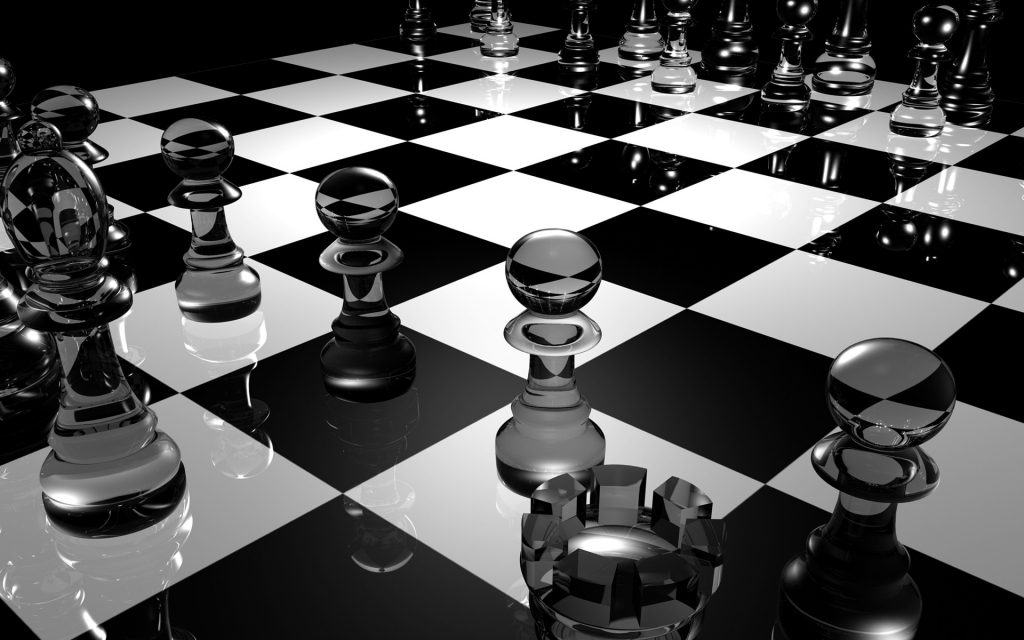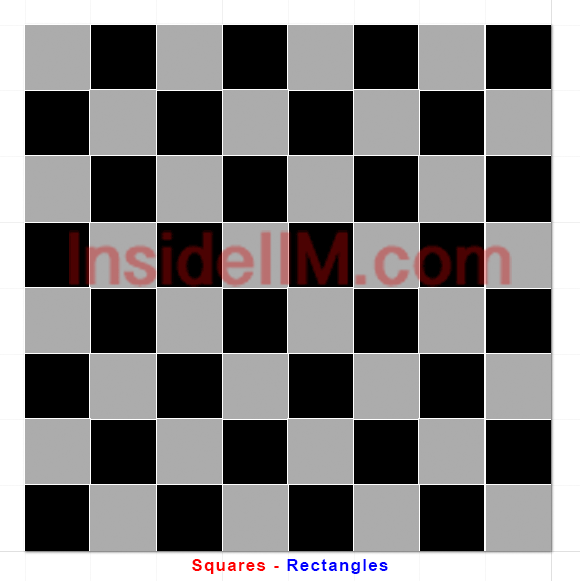# Do You Know The Number Of Squares And Rectangles In A ChessboardOften times in CAT exam, questions are asked on “How Many Squares Are There In A Chessboard?” or “How Many Rectangles Are There In A 8*8 Chess-board Which Are Not Squares?”, etc. These questions though look time confusing or difficult to some but are not if approached with right concept. Let’s have a look at the same!

Formula: Number of squares in a n*n Chessboard: 12 +22 +….+ n2  or [n*(n+1)*(2n+1)]/6

In a 2*2 chessboard, there are 5 squares (4 small squares, 1 big square)

=> 12 +22 = 5

So in a 8*8 chessboard, n=8
=> No. of squares = 12 + 2+ 32+…..+ 82
= [n*(n+1)*(2n+1)]/6 (summation formula)
= 204

Also, number of white and black squares in chessboard: 204/2 => 102 eachRectangles:

For an n*n chessboard, there are 13 + 2+ 33+…..+ n3 rectangles.

So for an 8*8 chessboard, there are, rectangles = 13 + 23+….+ 83

=> no. of rectangles = [{n2}*{(n+1)2}]/4

n=8, we get no. of rectangles = 1296

**No. of rectangles that are not squares in an 8*8 chessboard
=> 1296 – 204
= 1092

Likewise, it goes for 7*7, 6*6, 5*5, 4*4 or any other n*n matrix.

 Must Read: 15 Books to Read For CAT Preparation Must Read: CAT Preparation Strategy By IIM A|B|C Convert

## Chess Arrangement Problems

Independence problems

Given a certain chess piece (queen, rook, bishop, knight or king) find the maximum number of such pieces, which can be placed on a chess board so that none of the pieces attack each other.

The maximum number of independent kings on an 8×8 chessboard is 16, queens – 8, rooks – 8, bishops – 14, knights – 32.

Domination problems

In these problems it is requested to find a minimum number of pieces of the given kind and place them on a chess board in such a way, that all free squares of the board are attacked by at least one piece.

The minimal number of dominating kings is 9, queens – 5, rooks – 8, bishops – 8, knights – 12.

## What is the probability of getting two squares having a common side on a 8*8 chess board?

Method 1

No. of vertical lines in a chess board (not counting the external boundaries) is 7.
Each such vertical line is divided into 8 smaller parts.
Total no of such vertical smaller parts (vertical edges of small squares) = 8*7 => 56
Similarly, no of horizontal smaller edges = 56
total number of edges = 112.
Every single edge gives a unique combination of selecting squares that have a common edge.

Total number of ways of selecting two squares = 64C2.

Hence, probability = 112/64C2 = 0.0555

Method 2

There are three types of squares in a chess board.
Type 1: The four corners.
Type 2: The squares at the edges but not the corners = 24
Type 3: The squares which are not in the corners nor at the edge = 36

Type 1: For each square there are two possibilities. Total = 4*2 => 8
Type 2: For each square there are three possibilities. Total = 24*3 => 72
Type 3: For each square there are four possibilities. Total = 36*4 => 144

No of ways 1st square can be chosen = 64 ways.
no of ways 2nd one can be chosen = 63 ways.
Total = 64*63

Required Probability = 224/(64*63) = 0.055

If two squares are chosen at random on a chessboard, what is the probability that they have exactly one corner in common?

There are 3 types of squares, a different number of each type, and each with a different number of squares they share exactly one corner with:

1. Corners – 4 of them-shares exactly 1 corner with 1 square.
2. Edges – 24 of them-shares exactly 1 corner with 2 squares.
3. Other – 36 of them-shares exactly 1 corner with 4 squares.

Type 1: 4*1=4
Type 2: 24*2=48
Type 3: 36*4=144
Total = 196

Total Ways of choosing any 2 squares one after another = 64*63
Hence, required probability = 196/(64*63) = 0.486

Method 3

In the first and second row, there are 14 ways of selecting two (1 x 1) squares that have only one common corner.

Rows 2 and 3 will similarly have 14 squares that have only one common corner.

We can pick 7 such pairs of rows in a chess board viz., (1, 2), (2, 3), (3, 4), (4, 5), (5, 6), (6, 7), and (7,8)

Also Check:

## Indrajeet iQuanta

Indrajeet Singh, the CEO of iQuanta gave a new direction to the world of online teaching where the doubts gets cleared within seconds or minutes using the previously neglected medium, Facebook as his platform. He started iQuanta last year, and it delivered splendid results in its very first year with two 100%ilers, sixteen 99.6+ %ilers & around 30 final converts from IIM A,B,C and XLRI. iQuanta is mostly about unconventional methods and concepts which aren’t taught in conventional coaching centres and his free peer learning platform i.e. CAT Preparation group is 93k members strong and is highly active – https://www.facebook.com/groups/Rockthecat/

## 2 commentsRittu Paul

You have added the no. of possible outcomes for Type 1, Type 2, Type 3 cases but in the process arent we counting each selection two times like wen we consider a particular square say 1 sharing one side with square 2, we are again counting square 2 sharing the same side with square 1 so final answer should be half of what you are claiming. Please check and correct because this post might mislead 1000 of aspirants.Pramod Deshpande

Hi all, these kinds of questions how can they be answered if we don’t know about the chess game at ALL!!!
What are the examiners testing here?